# Multivariate Linear Regression From Scratch With Python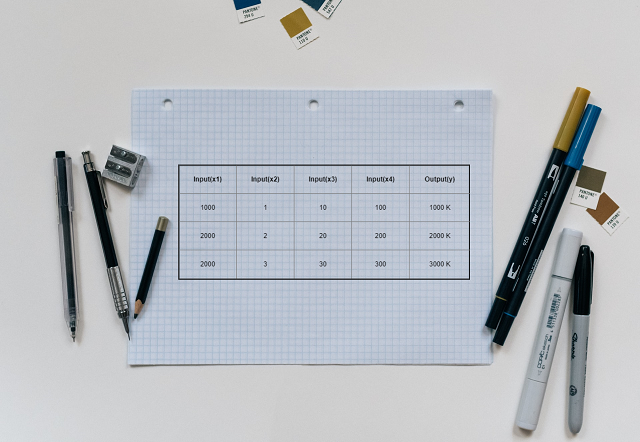In this tutorial we are going to cover linear regression with multiple input variables. We are going to use same model that we have created in Univariate Linear Regression tutorial. I would recommend to read Univariate Linear Regression tutorial first. We will define the hypothesis function with multiple variables and use gradient descent algorithm. We will also use plots for better visualization of inner workings of the model. At the end we will test our model using training data.

## Introduction

In case of multivariate linear regression output value is dependent on multiple input values. The relationship between input values, format of different input values and range of input values plays important role in linear model creation and prediction. I am using same notation and example data used in Andrew Ng’s Machine Learning course

## Hypothesis Function

Our hypothesis function for univariate linear regression was

``````h(x) = theta_0 + theta_1*x_1
where x_1 is only input value
``````

For multiple input value, hypothesis function will look like,

``````h(x) = theta_0 + theta_1 * x_1 + theta_2 * x_2 .....theat_n * x_n
where x_1, x_2...x_n are multiple input values
``````

If we consider the house price example then the factors affecting its price like house size, no of bedrooms, location etc are nothing but input variables of above hypothesis function.

## Cost Function

Our cost function remains same as used in Univariate linear regression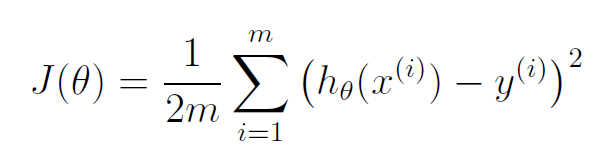For more details about cost function please refer ‘Create Cost Function’ section of Univariate Linear Regression

Gradient descent algorithm function format remains same as used in Univariate linear regression. But here we have to do it for all the theta values(no of theta values = no of features + 1).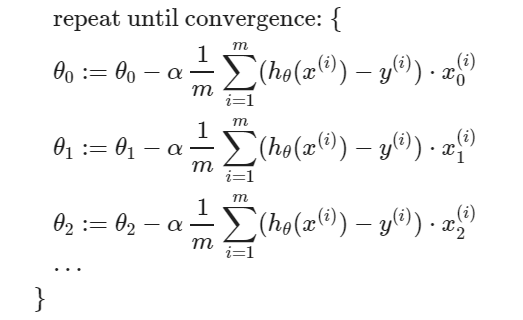# Python Code

## Notations used

• m = no of training examples (no of rows of feature matrix)
• n = no of features (no of columns of feature matrix)
• x’s = input variables / independent variables / features
• y’s = output variables / dependent variables / target

## Import the required libraries

• numpy : Numpy is the core library for scientific computing in Python. It is used for working with arrays and matrices.
• pandas: Used for data manipulation and analysis
• matplotlib : It’s plotting library, and we are going to use it for data visualization
``````import numpy as np
import pandas as pd
import matplotlib.pyplot as plt
``````

• We are going to use ‘multivariate_housing_prices_in_portlans_oregon.csv’ CSV file
• File contains three columns ‘size(in square feet)’, ‘number of bedrooms’ and ‘price’
``````df = pd.read_csv('https://raw.githubusercontent.com/satishgunjal/datasets/master/multivariate_housing_prices_in_portlans_oregon.csv')
df.head() # To get first n rows from the dataset default value of n is 5
``````
size(in square feet) number of bedrooms price
0 2104 3 399900
1 1600 3 329900
2 2400 3 369000
3 1416 2 232000
4 3000 4 539900
``````X = df.values[:, 0:2]  # get input values from first two columns
y = df.values[:, 2]  # get output values from last coulmn
m = len(y) # Number of training examples

print('Total no of training examples (m) = %s \n' %(m))

# Show only first 5 records
for i in range(5):
print('x =', X[i, ], ', y =', y[i])
``````
``````Total no of training examples (m) = 47

x = [2104    3] , y = 399900
x = [1600    3] , y = 329900
x = [2400    3] , y = 369000
x = [1416    2] , y = 232000
x = [3000    4] , y = 539900
``````

## Understand the data

• There are total 97 training examples (m= 97 or 97 no of rows)
• There are two features (two columns of feature and one of label/target/y)
• Total no of features (n) = 2 (Later we will add column of ones(x_0) to make it 3)

### Feature Normalization

• As you can notice size of the house and no of bedrooms are not in same range(house sizes are about 1000 times the number of bedrooms). This will have negative impact on gradient descent algorithm performance.
• In gradient descent algorithm we calculate the cost for every step. And if our input values differ by order of magnitude then results after every gradient descent step will also vary a lot.
• We can avoid this by changing the range of our input variables.
• We use below techniques to change the range of input variables
• Feature Scaling
• Mean Normalization
• Feature Scaling: In feature scaling we divide the input value by range(max - min) of input variable. By this technique we get new range of just 1.

``````  x1 = x1 / s1
where,
x1 = input variable
s1 = range

``````
• Mean Normalization: In mean normalization we subtract the average value from the input variable and then divide it by range(max - min) or by standard deviation of input variable.

``````  x1 = (x1 - mu1)/s1
where,
x1 = input variable
mu1 = average value
s1 = range or standard deviation
``````

Lets create function to normalize the value of input variable

``````def feature_normalize(X):
"""
Normalizes the features(input variables) in X.

Parameters
----------
X : n dimensional array (matrix), shape (n_samples, n_features)
Features(input varibale) to be normalized.

Returns
-------
X_norm : n dimensional array (matrix), shape (n_samples, n_features)
A normalized version of X.
mu : n dimensional array (matrix), shape (n_features,)
The mean value.
sigma : n dimensional array (matrix), shape (n_features,)
The standard deviation.
"""
#Note here we need mean of indivdual column here, hence axis = 0
mu = np.mean(X, axis = 0)
# Notice the parameter ddof (Delta Degrees of Freedom)  value is 1
sigma = np.std(X, axis= 0, ddof = 1)  # Standard deviation (can also use range)
X_norm = (X - mu)/sigma
return X_norm, mu, sigma
``````
``````X, mu, sigma = feature_normalize(X)

print('mu= ', mu)
print('sigma= ', sigma)
print('X_norm= ', X[:5])
``````
``````mu=  [2000.68085106    3.17021277]
sigma=  [7.94702354e+02 7.60981887e-01]
X_norm=  [[ 0.13000987 -0.22367519]
[-0.50418984 -0.22367519]
[ 0.50247636 -0.22367519]
[-0.73572306 -1.53776691]
[ 1.25747602  1.09041654]]
``````

Note: New mean or avearage value of normalized X feature is 0

`````` mu_testing = np.mean(X, axis = 0) # mean
mu_testing
``````
``````array([3.77948264e-17, 2.74603035e-16])
``````

Note: New range or standard deviation of normalized X feature is 1

``````
sigma_testing = np.std(X, axis = 0, ddof = 1) # mean
sigma_testing
``````
``````array([1., 1.])
``````
``````# Lets use hstack() function from numpy to add column of ones to X feature
# This will be our final X matrix (feature matrix)
X = np.hstack((np.ones((m,1)), X))
X[:5]
``````
``````array([[ 1.        ,  0.13000987, -0.22367519],
[ 1.        , -0.50418984, -0.22367519],
[ 1.        ,  0.50247636, -0.22367519],
[ 1.        , -0.73572306, -1.53776691],
[ 1.        ,  1.25747602,  1.09041654]])
``````

Note above, we have added column of ones to X so final dimension of X is mxn i.e 97x3

## Compute Cost

• function definition is same as used in Univariate Linear Regression
• numpy.dot() this function returns the dot product of two arrays. For 2-D vectors, it is the equivalent to matrix multiplication
• numpy.subtract() this function perform the element wise subtraction
• numpy.square() this function perform the element wise square
``````def compute_cost(X, y, theta):
"""
Compute the cost of a particular choice of theta for linear regression.

Input Parameters
----------------
X : 2D array where each row represent the training example and each column represent the feature ndarray. Dimension(m x n)
m= number of training examples
n= number of features (including X_0 column of ones)
y : 1D array of labels/target value for each traing example. dimension(1 x m)

theta : 1D array of fitting parameters or weights. Dimension (1 x n)

Output Parameters
-----------------
J : Scalar value.
"""
predictions = X.dot(theta)
#print('predictions= ', predictions[:5])
errors = np.subtract(predictions, y)
#print('errors= ', errors[:5])
sqrErrors = np.square(errors)
#print('sqrErrors= ', sqrErrors[:5])
#J = 1 / (2 * m) * np.sum(sqrErrors)
# OR
# We can merge 'square' and 'sum' into one by taking the transpose of matrix 'errors' and taking dot product with itself
J = 1/(2 * m) * errors.T.dot(errors)

return J
``````

``````def gradient_descent(X, y, theta, alpha, iterations):
"""
Compute cost for linear regression.

Input Parameters
----------------
X : 2D array where each row represent the training example and each column represent the feature ndarray. Dimension(m x n)
m= number of training examples
n= number of features (including X_0 column of ones)
y : 1D array of labels/target value for each traing example. dimension(m x 1)
theta : 1D array of fitting parameters or weights. Dimension (1 x n)
alpha : Learning rate. Scalar value
iterations: No of iterations. Scalar value.

Output Parameters
-----------------
theta : Final Value. 1D array of fitting parameters or weights. Dimension (1 x n)
cost_history: Conatins value of cost for each iteration. 1D array. Dimansion(m x 1)
"""
cost_history = np.zeros(iterations)

for i in range(iterations):
predictions = X.dot(theta)
#print('predictions= ', predictions[:5])
errors = np.subtract(predictions, y)
#print('errors= ', errors[:5])
sum_delta = (alpha / m) * X.transpose().dot(errors);
#print('sum_delta= ', sum_delta[:5])
theta = theta - sum_delta;

cost_history[i] = compute_cost(X, y, theta)

return theta, cost_history
``````

Lets update the gradient descent learning parameters alpha and no of iterations

``````# We need theta parameter for every input variable. since we have three input variable including X_0 (column of ones)
theta = np.zeros(3)
iterations = 400;
alpha = 0.15;
``````
``````theta, cost_history = gradient_descent(X, y, theta, alpha, iterations)
print('Final value of theta =', theta)
print('First 5 values from cost_history =', cost_history[:5])
print('Last 5 values from cost_history =', cost_history[-5 :])
``````
``````Final value of theta = [340412.65957447 110631.0502787   -6649.47427067]
First 5 values from cost_history = [4.76541088e+10 3.48804679e+10 2.57542477e+10 1.92146908e+10
1.45159772e+10]
Last 5 values from cost_history = [2.04328005e+09 2.04328005e+09 2.04328005e+09 2.04328005e+09
2.04328005e+09]
``````

Note: ‘cost_history’ contains the values of cost for every step of gradient descent, and its value should decrease for every step of gradient descent

## Visualization

• cost_history contains the values of cost for every iteration performed during batch gradient descent
• If all our parameters are correct then cost should reduce for every iteration(step)
• Lets plot the values of cost against no of iterations to visualize the performance of the Gradient Descent Algorithm
``````import matplotlib.pyplot as plt
plt.plot(range(1, iterations +1), cost_history, color ='blue')
plt.rcParams["figure.figsize"] = (10,6)
plt.grid()
plt.xlabel("Number of iterations")
plt.ylabel("cost (J)")
``````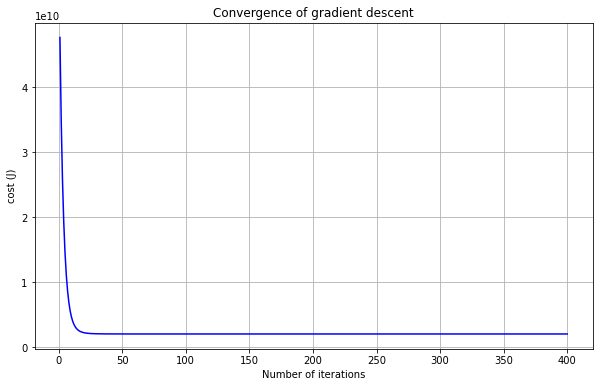Note that curve flattened out at around 20th iteration and ramains same affter that. This is the indication of convergence of gradient descent

## Effect Of Learning Rate On Convergence

• To check the effect of learning rate on convergence lets store the cost history for different learning rate and plot convergence plot for better visualization
• Notice the changes in the convergence curves as the learning rate changes.
• With a small learning rate(alpha = 0.005, purple line), gradient descent takes a very long time to converge to the optimal value.
• As we increase the alpha value, slope becomes sharp and gradient descent will take less time to converge
• But if the value of learning rate(alpha = 1.32, brown line) is too large then gradient descent may not decrease on every iteration, may even diverge
``````iterations = 400;
theta = np.zeros(3)

alpha = 0.005;
theta_1, cost_history_1 = gradient_descent(X, y, theta, alpha, iterations)

alpha = 0.01;
theta_2, cost_history_2 = gradient_descent(X, y, theta, alpha, iterations)

alpha = 0.02;
theta_3, cost_history_3 = gradient_descent(X, y, theta, alpha, iterations)

alpha = 0.03;
theta_4, cost_history_4 = gradient_descent(X, y, theta, alpha, iterations)

alpha = 0.15;
theta_5, cost_history_5 = gradient_descent(X, y, theta, alpha, iterations)

plt.plot(range(1, iterations +1), cost_history_1, color ='purple', label = 'alpha = 0.005')
plt.plot(range(1, iterations +1), cost_history_2, color ='red', label = 'alpha = 0.01')
plt.plot(range(1, iterations +1), cost_history_3, color ='green', label = 'alpha = 0.02')
plt.plot(range(1, iterations +1), cost_history_4, color ='yellow', label = 'alpha = 0.03')
plt.plot(range(1, iterations +1), cost_history_5, color ='blue', label = 'alpha = 0.15')

plt.rcParams["figure.figsize"] = (10,6)
plt.grid()
plt.xlabel("Number of iterations")
plt.ylabel("cost (J)")
plt.title("Effect of Learning Rate On Convergence of Gradient Descent")
plt.legend()

``````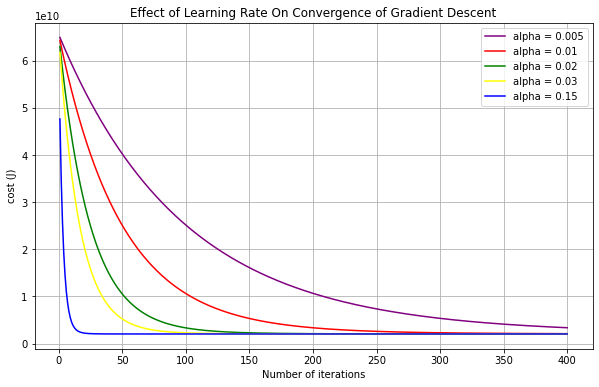``````iterations = 100;
theta = np.zeros(3)

alpha = 1.32;
theta_6, cost_history_6 = gradient_descent(X, y, theta, alpha, iterations)

plt.plot(range(1, iterations +1), cost_history_6, color ='brown')
plt.rcParams["figure.figsize"] = (10,6)
plt.grid()
plt.xlabel("Number of iterations")
plt.ylabel("cost (J)")
plt.title("Effect of Large Learning Rate On Convergence of Gradient Descent")
``````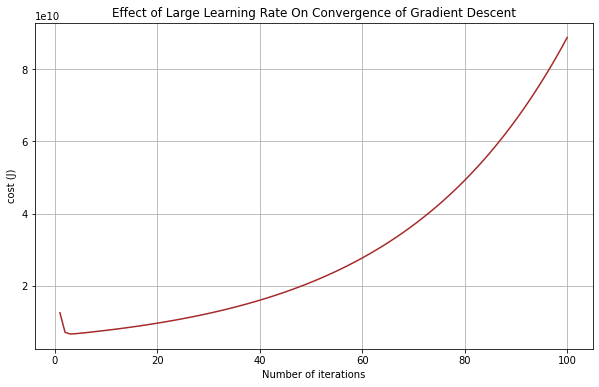## Testing the model

• Question: Estimate the price of a 1650 sq-ft, 3-bedroom house

• Remember that we have normalized the data for in order to use the gradient descent algorithm. So we have to also normalize the given input data before any prediction
• Also we have to add column of ones to input data the way we have added to input feature X
``````normalize_test_data = ((np.array([1650, 3]) - mu) / sigma)
normalize_test_data = np.hstack((np.ones(1), normalize_test_data))
price = normalize_test_data.dot(theta)
print('Predicted price of a 1650 sq-ft, 3 br house:', price)
``````
``````Predicted price of a 1650 sq-ft, 3 br house: 293081.46433492796
``````

## Conclusion

This concludes our multivariate linear regression. Now we know how to perform the feature normalization and linear regression when there are multiple input variables. In next tutorial we will use scikit-learn linear model to perform the linear regression.

## Learning Path for DP-900 Microsoft Azure Data Fundamentals Certification

Learning path to gain necessary skills and to clear the Azure Data Fundamentals Certification. This certification is intended for candidates beginning to wor...

## Learning Path for AI-900 Microsoft Azure AI Fundamentals Certification

Learning path to gain necessary skills and to clear the Azure AI Fundamentals Certification. This certification is intended for candidates with both technica...

## ANN Model to Classify Images

In this guide we are going to create and train the neural network model to classify the clothing images. We will use TensorFlow deep learning framework along...

## Introduction to NLP

In short NLP is an AI technique used to do text analysis. Whenever we have lots of text data to analyze we can use NLP. Apart from text analysis, NLP also us...

## K Fold Cross Validation

There are multiple ways to split the data for model training and testing, in this article we are going to cover K Fold and Stratified K Fold cross validation...

## K-Means Clustering

K-Means clustering is most commonly used unsupervised learning algorithm to find groups in unlabeled data. Here K represents the number of groups or clusters...

## Time Series Analysis and Forecasting

Any data recorded with some fixed interval of time is called as time series data. This fixed interval can be hourly, daily, monthly or yearly. Objective of t...

## Support Vector Machines

Support vector machines is one of the most powerful ‘Black Box’ machine learning algorithm. It belongs to the family of supervised learning algorithm. Used t...

## Random Forest

Random forest is supervised learning algorithm and can be used to solve classification and regression problems. Unlike decision tree random forest fits multi...

## Decision Tree

Decision tree explained using classification and regression example. The objective of decision tree is to split the data in such a way that at the end we hav...

## Agile Scrum Framework

This tutorial covers basic Agile principles and use of Scrum framework in software development projects.

## Underfitting & Overfitting

Main objective of any machine learning model is to generalize the learning based on training data, so that it will be able to do predictions accurately on un...

## Multiclass Logistic Regression Using Sklearn

In this study we are going to use the Linear Model from Sklearn library to perform Multi class Logistic Regression. We are going to use handwritten digit’s d...

## Binary Logistic Regression Using Sklearn

In this tutorial we are going to use the Logistic Model from Sklearn library. We are also going to use the same test data used in Logistic Regression From Sc...

## Logistic Regression From Scratch With Python

This tutorial covers basic concepts of logistic regression. I will explain the process of creating a model right from hypothesis function to algorithm. We wi...

## Train Test Split

In this tutorial we are going to study about train, test data split. We will use sklearn library to do the data split.

## One Hot Encoding

In this tutorial we are going to study about One Hot Encoding. We will also use pandas and sklearn libraries to convert categorical data into numeric data.

## Multivariate Linear Regression Using Scikit Learn

In this tutorial we are going to use the Linear Models from Sklearn library. Scikit-learn is one of the most popular open source machine learning library for...

## Univariate Linear Regression Using Scikit Learn

In this tutorial we are going to use the Linear Models from Sklearn library. Scikit-learn is one of the most popular open source machine learning library for...

## Multivariate Linear Regression From Scratch With Python

In this tutorial we are going to cover linear regression with multiple input variables. We are going to use same model that we have created in Univariate Lin...